### Extracting ROM constants from the 8087 math coprocessor's die

Intel introduced the 8087 chip in 1980 to improve floating-point performance on the 8086 and 8088 processors, and it was used with the original IBM PC. Since early microprocessors operated only on integers, arithmetic with floating-point numbers was slow and transcendental operations such as arctangent or logarithms were even worse. Adding the 8087 co-processor chip to a system made floating-point operations up to 100 times faster.

I opened up an 8087 chip and took photos with a microscope. The photo below shows the chip's tiny silicon die. Around the edges of the chip, tiny bond wires connect the chip to the 40 external pins. The labels show the main functional blocks, based on my reverse engineering. By examining the chip closely, various constants can be read out of the chip's ROM, numbers such as pi that the chip uses in its calculations.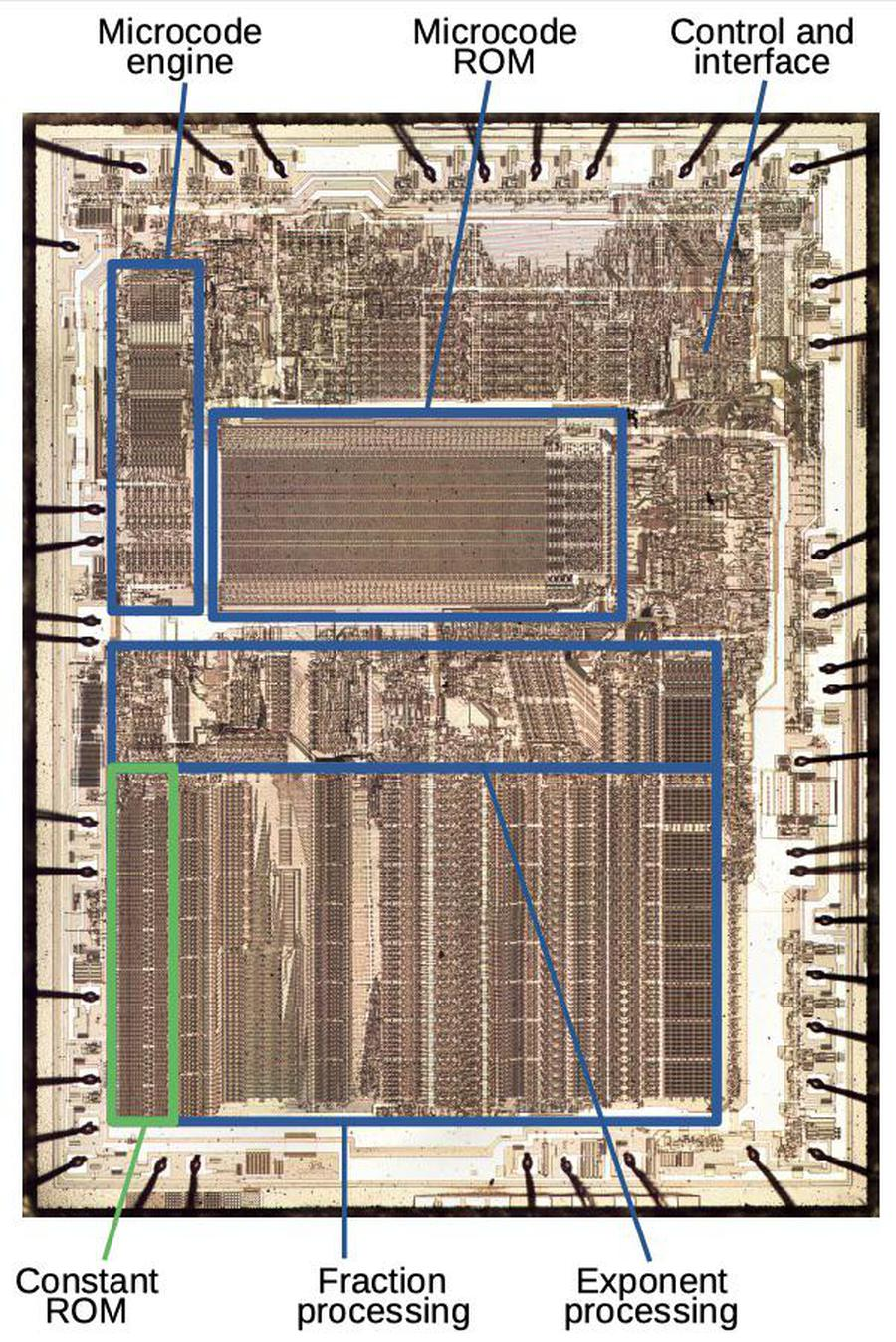Die of the Intel 8087 floating point unit chip, with main functional blocks labeled. The constant ROM is outlined in green. Click for a larger image.

The top half of the chip contains the control circuitry. Performing a floating-point instruction might require 1000 steps; the 8087 used microcode to specify these steps. The die photo above shows the "engine" that ran the microcode program; it is basically a simple CPU. Next to it is the large ROM that holds the microcode.

The bottom half of the die holds the circuitry that processes floating-point numbers. A floating-point number consists of a fraction (also called significand or mantissa), an exponent, and a sign bit. (For a base-10 analogy, in the number 6.02×1023, 6.02 is the fraction and 23 is the exponent.) The chip has separate circuitry to process the fraction and the exponent in parallel. The fraction processing circuitry supports 67-bit values, a 64-bit fraction with three extra bits for accuracy. From left to right, the fraction circuitry consists of a constant ROM, a shifter, adder/subtracters, and the register stack. The constant ROM (highlighted in green) is the subject of this post.

The 8087 operated as a co-processor with the 8086 processor. When the 8086 encountered a special floating-point instruction, the processor ignored it and let the 8087 execute the instruction in parallel.1 I won't explain in detail how the 8087 works internally, but as an overview, floating-point operations are implemented using integer adds/subtracts and shifts. To add or subtract two floating-point numbers, the 8087 shifts the numbers until the binary points (i.e. the decimal points but in binary) line up, and then adds or subtracts the fraction. Multiplication, division, and square root are performed through repeated shifts and adds or subtracts. Transcendental operations (tan, arctan, log, power) use CORDIC algorithms, which use shifts and adds of special constants for efficient computation.

## Implementation of the ROM

This post describes the ROM that holds constants (not to be confused with the larger, four-level microcode ROM.2) The constant ROM holds the constants (such as pi, ln(2), and sqrt(2)) that the 8087 needs for its computations. The photo below shows part of the constant ROM. The metal layer has been removed to show the silicon underneath. The pinkish regions are silicon doped to have different properties, while the reddish and greenish lines are polysilicon, a special type of silicon wiring layered on top. Note the regular grid structure of the ROM. The ROM consists of two columns of transistors, holding the bits. To explain how the ROM works, I'll start by explaining how a transistor works.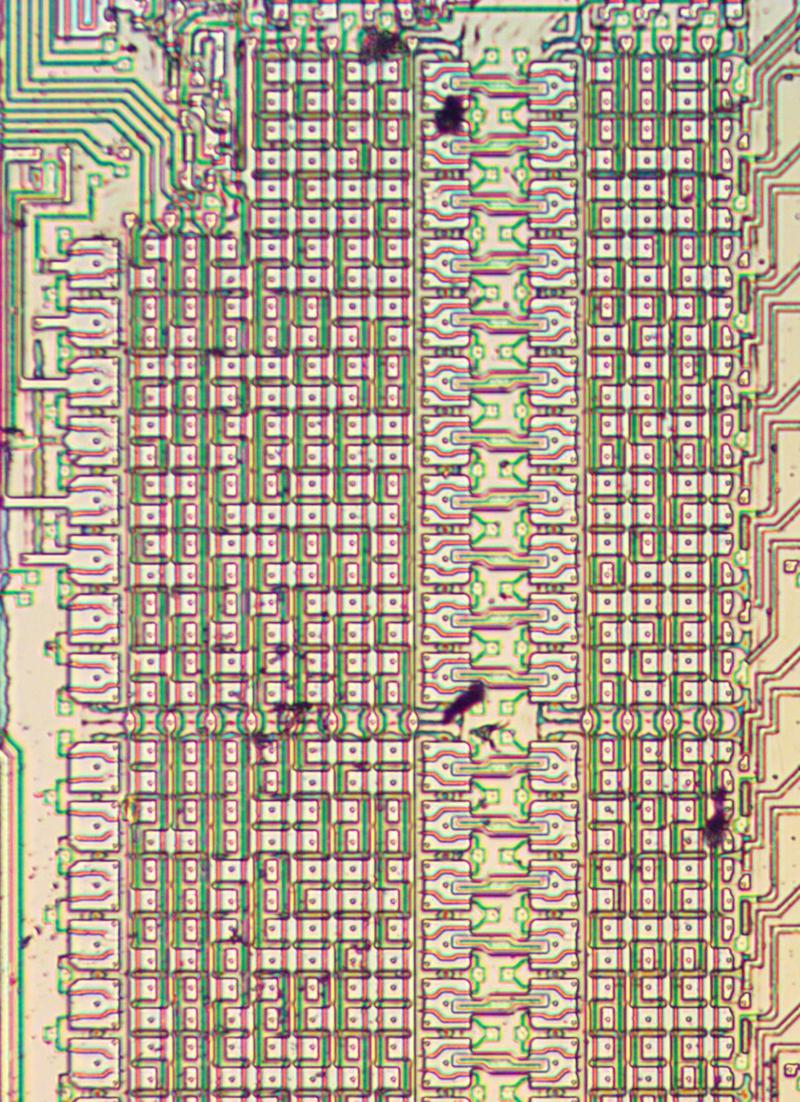Part of the constant ROM, with the metal layer removed. The three columns of larger transistors are used to select between rows.

High-density integrated circuits in the 1970s were usually built from a type of transistor known as NMOS. (Modern computers are built from CMOS, which consists of NMOS transistors along with opposite-polarity PMOS transistors.) The diagram below shows the structure of an NMOS transistor. An integrated circuit is constructed from a silicon substrate, with transistors built on it. Regions of the silicon are doped with impurities to create "diffusion" regions with desired electrical properties. The transistor can be viewed as a switch, allowing current to flow between two diffusion regions called the source and drain. The transistor is controlled by the gate, made of a special type of silicon called polysilicon. Applying voltage to the gate lets current flow between the source and drain, which is otherwise blocked. The die of the 8087 is fairly complex, with about 40,000 of these transistors.3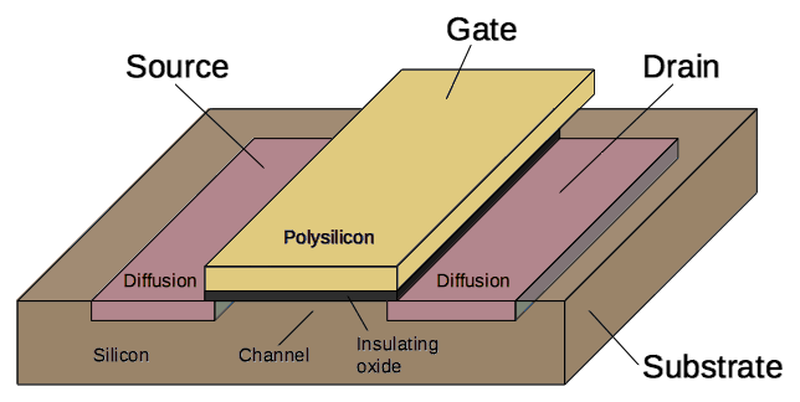Structure of a MOSFET as implemented in an integrated circuit.

Zooming in on the ROM shows the individual transistors. The pinkish regions are the doped silicon, forming transistor sources and drains. The vertical polysilicon select lines form the gates of the transistors. The indicated silicon regions are connected to ground, pulling one side of each transistor low. The circles are connections called vias between the silicon and the metal lines above. (The metal lines have been removed; the orange line shows the position of one.)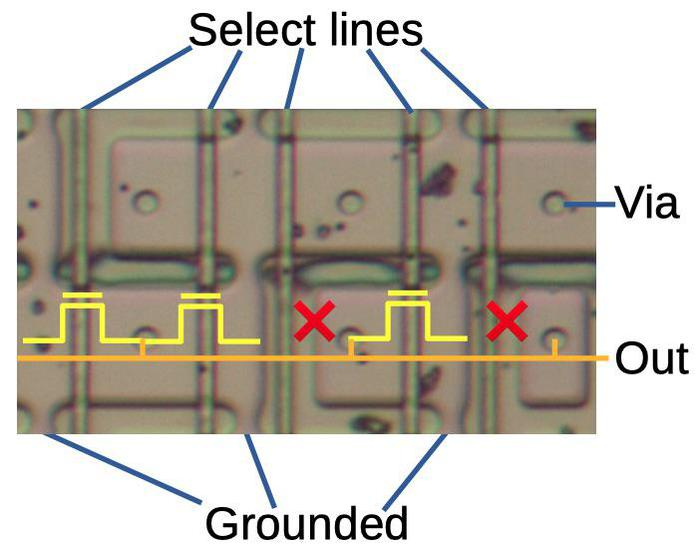A portion of the constant ROM. Each select line selects a particular constant. Transistors are indicated by the yellow symbols. An X indicates a missing transistor, corresponding to a 0 bit. The orange line indicates the position of a metal wire. (The metal layer was dissolved for this picture.)

The important feature of the ROM is that some of the transistors are missing, the first one in the upper row, and two marked with X in the lower row. Bits are programmed into the ROM by changing the silicon doping pattern, creating transistors or leaving insulating regions. Each transistor or missing transistor represents one bit. When a select line is activated, all the transistors in that column will turn on, pulling the corresponding output lines low. But if the transistor is missing from a selected position, the corresponding output line will remain high. Thus, a value is read from the ROM by activating a select line, reading that ROM value onto the output lines.

# Contents of the ROM

The constant ROM has 134 rows of 21 columns.5 Under a microscope, the bit pattern of the ROM is visible and can be extracted.4 How to interpret the raw bits is not obvious, though. The first question is if a transistor (versus a gap) indicates a 0 or a 1. (It turns out that a transistor indicates a 1 bit.) The next issue is how to map the 134×21 grid of bits into values.6

The chip's data path consists of 67 horizontal rows, so it seemed pretty clear that the 134 rows in the ROM corresponded to two sets of 67-bit constants. I extracted one set of constants for the odd rows and one for the even rows, but the values didn't make any sense. After more thought, I determined that the rows do not alternate but are arranged in a repeating "ABBA" pattern.7 Using this pattern yielded a bunch of recognizable constants, including pi and 1. Bits from those constants are shown in the diagram below. (In this photo, a 1 bit appears as a green stripe, while a 0 bit appears as a red stripe.) In binary, pi is 11.001001... and this value is visible in the upper labeled bits. The bottom value is the constant 1.8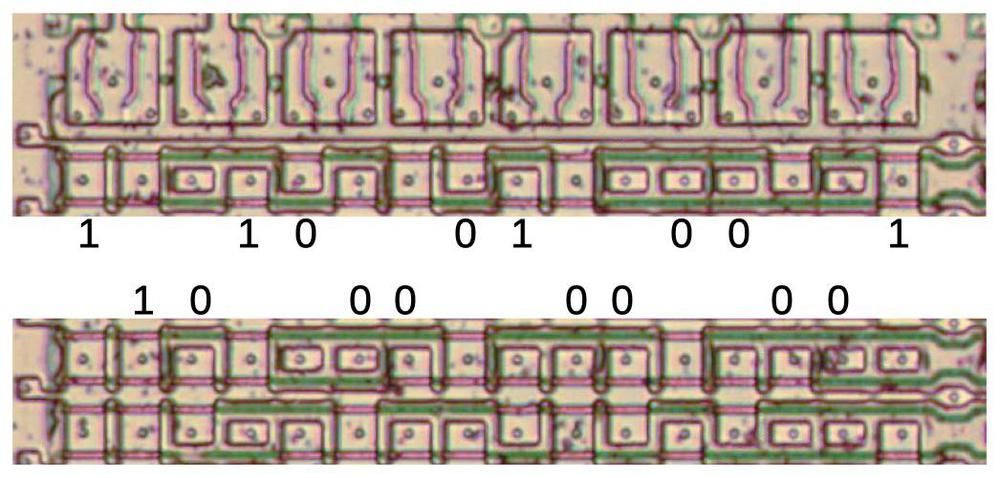Bit values labeled in the constant ROM. The top bits are the first part of pi, while the lower bits are the constant 1, This diagram has been rotated 90 degrees compared to the other diagrams. The unlabeled bits form other constants.

The next difficulty in interpretation is that this ROM holds just the fractional parts of the numbers, not the exponents. (I haven't found the separate exponent ROM yet.) I experimented with various exponents until I got values that were sensible numbers. Some were straightforward: for instance, the constant 1.204120 yielded log10(2) when the exponent 2-2 was used. Others were harder,9 such as 1.734723. Eventually, I figured out that 1.734723×259 is 1018.10

The complete table of constants is in the footnotes.11 Physically, the constants are arranged in three groups. The first group is values that the user can load (1, pi, log210, log2e, log102, and ln 2)12 along with values used internally (1018, ln(2)/3, 3*log2(e), log2(e), and sqrt(2)). The second group is sixteen arctan constants, and the third is fourteen log2 constants. The last two groups of constants are used to compute transcendental functions using the CORDIC algorithm, which I will discuss next.

## The CORDIC algorithms

The constants in the ROM reveal some details about the algorithms used by the 8087. The ROM contains 16 arctangent values, the arctans of 2-n. It also contains 14 log values, the base-2 logs of (1+2-n). These may seem like unusual values, but they are used in an efficient algorithm called CORDIC, which was invented in 1958.

The basic idea of CORDIC is to compute tangent and arctangent by breaking down an angle into smaller angles, and rotating a vector by these angles. The trick is that by carefully choosing the smaller angles, each rotation can be computed with efficient shifts and adds instead of trig functions. Specifically, suppose we want to find tan(z). We can break z into a sum of smaller angles: z ≈ {atan(2-1) or 0} + {atan(2-2) or 0} + {atan(2-3) or 0} + ... + {atan(2-16) or 0}. Now, rotating a vector by, say atan(2-2), can be done by multiplying by 2-2 and adding. The key thing is that multiplying by 2-2 is just a fast bit shift. Putting this all together, computing tan(z) can be done by comparing z with the atan constants, and then doing 16 cycles of additions and shifts, which are fast to perform in hardware.13 To make the algorithm work, the atan constants are precomputed and stored in the constant ROM.14

Computing the base-2 log and base-2 exponential also use CORDIC algorithms, with the associated logarithmic constants. The key observation is that multiplying by (1 + 2-n) can be done quickly with a shift and addition. By multiplying one side of the equation by the sequence of values, and adding the corresponding log constants to the other side, the log or exponential can be computed.15

The 8087's support for transcendental functions is more limited than you might expect. It only supports tangent and arctangent, not sine or cosine; the user must apply trig identities to compute sine or cosine. Logs and exponentials only support base 2; for base 10 or base e, the user must apply the appropriate scale factor. At the time, the 8087 pushed the limits of what could fit on a chip, so the instruction set was limited to the essentials.

## Conclusion

The 8087 is a complex chip and at first it looks like a hopeless maze of circuitry. But much of it can be understood with careful study. It contains 42 constants in a ROM, and the values of these constants can be extracted under a microscope. Some of the constants (such as pi) are expected, while others (such as ln(2)/3) are more puzzling. Many of the constants are used for computing the tangent, arctangent, log, and power functions, using fast CORDIC algorithms.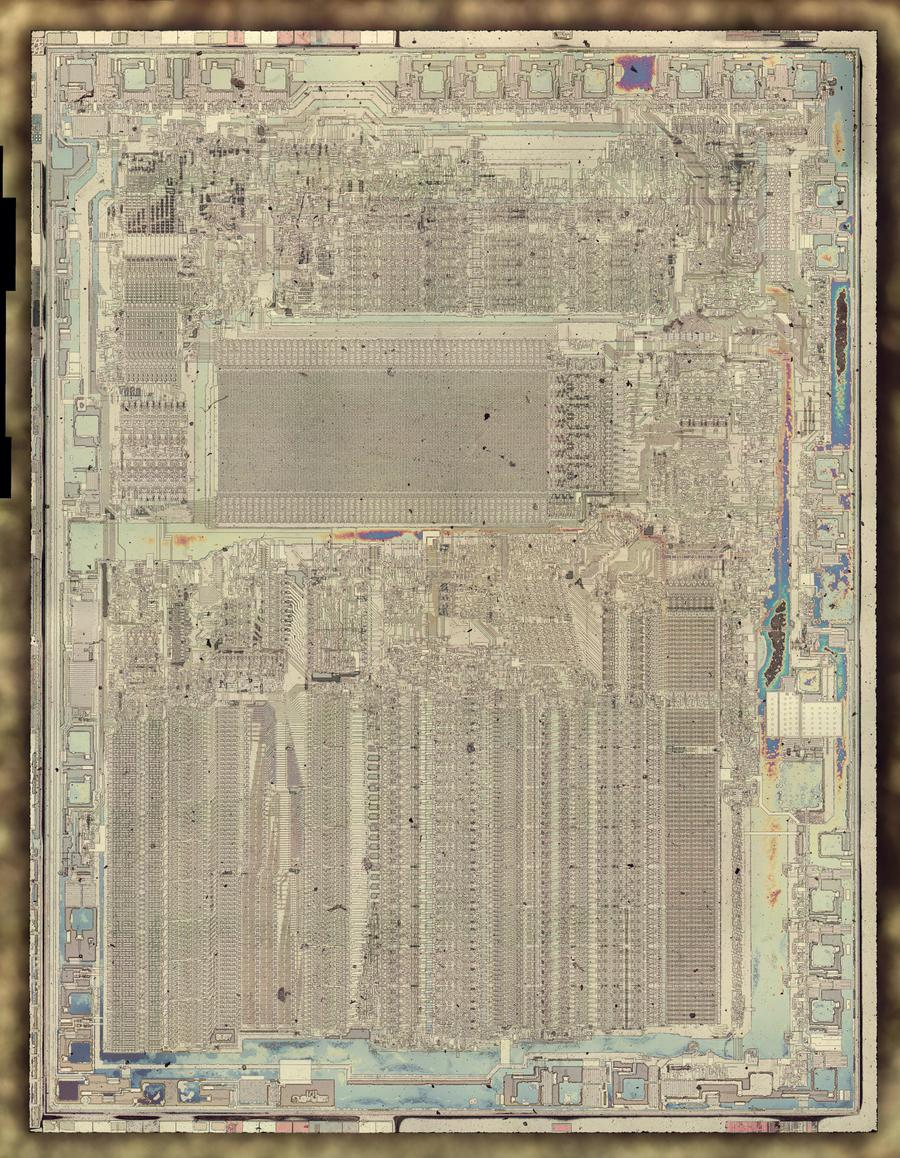Die photo of the 8087 with the metal layer removed. Click for a larger image.

Even though Intel's 8087 floating point unit chip was introduced 40 years ago, it still has a large influence today. It spawned the IEEE 754 floating-point standard used for most modern floating-point arithmetic, and the 8087's instructions remain a part of the x86 processors used in most computers.

For more information on the 8087, see my other articles: the two-bit-per-transistor ROM and the substrate bias generator. I announce my latest blog posts on Twitter, so follow me @kenshirriff for future articles. I also have an RSS feed.

## Notes and references

1. The interaction between the 8086 processor and the 8087 floating point unit is somewhat tricky; I'll discuss some highlights. The simplified view is that the 8087 watches the 8086's instruction stream, and executes any instructions that are 8087 instructions. The complication is that the 8086 has an instruction prefetch buffer, so the instruction being fetched isn't the one being executed. Thus, the 8087 duplicates the 8086's prefetch buffer (or the 8088's smaller prefetch buffer), so it knows that the 8086 is doing. (A Twitter thread discusses this in detail.) Another complication is the complex addressing modes used by the 8086, which use registers inside the 8086. The 8087 can't perform these addressing modes since it doesn't have access to the 8086 registers. Instead, when the 8086 sees an 8087 instruction, it does a memory fetch from the addressed location and ignores the result. Meanwhile, the 8087 grabs the address off the bus so it can use the address if it needs it. If there is no 8087 present, you might expect a trap, but that's not what happens. Instead, for a system without an 8087, the linker rewrites the 8087 instructions, replacing them with subroutine calls to the emulation library.

2. The 8087's microcode ROM is built with an unusual technique that stores two bits per transistor. It does this by using three different transistor sizes or no transistor in each position. The four possibilities at each position represent two bits. This complex technique was necessary in order to fit the large ROM onto the 8087 die. I wrote a blog post with more details. The constant ROM, in comparison, is built using standard techniques.

3. Sources provide inconsistent values for the number of transistors in the 8087: Intel claims 40,000 transistors while Wikipedia claims 45,000. The discrepancy could be due to different ways of counting transistors. In particular, since the number of transistors in a ROM, PLA or similar structure depends on the data stored in it, sources often count "potential" transistors rather than the number of physical transistors. Other discrepancies can be due to whether or not pull-up transistors are counted and if high-current drivers are counted as multiple transistors in parallel or one large transistor.

4. Instead of copying bits from the ROM by hand, I made a simple JavaScript program to help me read out the ROM. I clicked on the ROM image to indicate each transistor, and the program produced the corresponding pattern of 0's and 1's.

5. The ROM has 134 rows of 21 bits, except there is a 6×6 chunk missing from the upper left. Thus, the physical size is of the constant ROM is 2946 bits.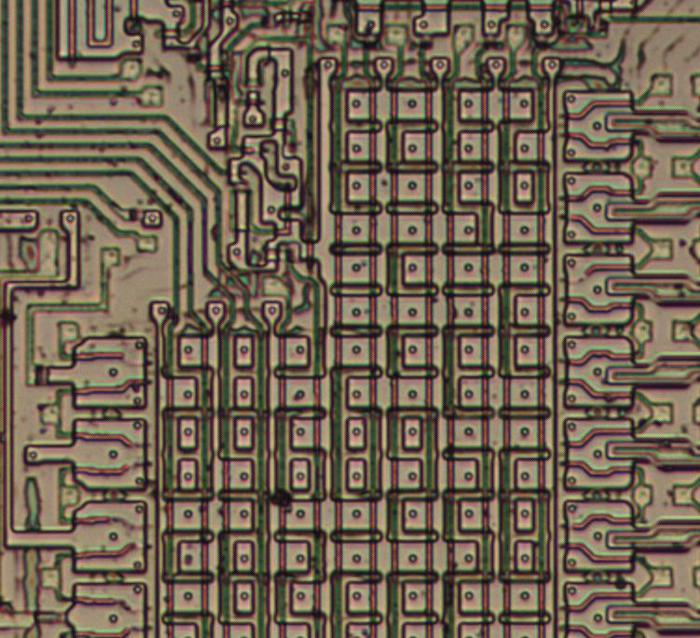The upper-left corner of the constant ROM, showing the missing 6×6 section.

Because of the ROM layout, this missing section means that the first 12 constants are 64 bits long, rather than 67 bits. These are the non-CORDIC constants, which apparently don't require the extra bits for accuracy.

6. There are two ways to determine the encoding of the bits. The first is to trace out the circuitry that reads from the ROM and examine how the data is used. The second is to look for patterns in the raw data, and determine what makes sense for an encoding. Since the 8087 is very complex, I wanted to avoid a full reverse-engineering to understand the constants and I used the second approach.

7. The organization of the rows follows the pattern ABBAABBAABBA..., where "A" rows hold bits for one set of constants and "B" rows hold bits for the second set of constants. This layout was probably used instead of alternating rows ("ABAB") because one connection can drive two neighboring selection transistors. That is, each "AA" or "BB" group can be selected with one wire.

8. A bit more trial-and-error was necessary to pull the values out of the ROM. I determined three key factors. First, the bits started at the bottom of the ROM, going up. Second, a transistor indicated a 1, rather than a 0. Third, the constants did not have an implicit 1 bit at the beginning. (In other words, the constant format does not match the external data format used by the 8087.)

9. Some of the exponents were tricky to determine. I used brute force for some of them, seeing if any exponent would yield the log or power of some number. One of the hardest numbers to figure out was ln(2)/3; I'm not sure why this value is important.

10. Why does the 8087 contain the constant 1018? Probably because the 8087 supports a packed BCD datatype holding 18 digits, so it can hold up to 1018

11. The following table summarizes the contents of the constant ROM. The "meaning" column is my interpretation of the number.

ConstantDecimal valueMeaning
1.204120×2-20.3010300log10(2)
1.386294×2-10.6931472ln(2)
1.442695×201.4426950log2(e)
1.570796×213.1415927Pi
1.000000×201.00000001
1.660964×213.3219281log2(10)
1.734723×2591.000e+181018
1.734723×2591.000e+181018
1.848392×2-30.2310491ln(2)/3
1.082021×224.32808513*log2(e)
1.442695×201.4426950log2(e)
1.414214×201.4142136sqrt(2)
1.570796×2-10.7853982atan(20)
1.854590×2-20.4636476atan(2-1)
2.000000×2-150.0000610atan(2-14)
2.000000×2-160.0000305atan(2-15)
1.959829×2-30.2449787atan(2-2)
1.989680×2-40.1243550atan(2-3)
2.000000×2-130.0002441atan(2-12)
2.000000×2-140.0001221atan(2-13)
1.997402×2-50.0624188atan(2-4)
1.999349×2-60.0312398atan(2-5)
1.999999×2-110.0009766atan(2-10)
2.000000×2-120.0004883atan(2-11)
1.999837×2-70.0156237atan(2-6)
1.999959×2-80.0078123atan(2-7)
1.999990×2-90.0039062atan(2-8)
1.999997×2-100.0019531atan(2-9)
1.441288×2-90.0028150log2(1+2-9)
1.439885×2-80.0056245log2(1+2-8)
1.437089×2-70.0112273log2(1+2-7)
1.431540×2-60.0223678log2(1+2-6)
1.442343×2-110.0007043log2(1+2-11)
1.441991×2-100.0014082log2(1+2-10)
1.420612×2-50.0443941log2(1+2-5)
1.399405×2-40.0874628log2(1+2-4)
1.442607×2-130.0001761log2(1+2-13)
1.442519×2-120.0003522log2(1+2-12)
1.359400×2-30.1699250log2(1+2-3)
1.287712×2-20.3219281log2(1+2-2)
1.442673×2-150.0000440log2(1+2-15)
1.442651×2-140.0000881log2(1+2-14)

It's clear from the CORDIC constants that the values in the ROM are not physically stored in order, i.e. sequential rows are not addressed in order. I'm not sure why 1018 appears twice; probably one exponent is different. The binary exponents are not in the ROM that I examined, so I had to estimate them.

12. The 8087 provides seven instructions to load constants directly. The instructions `FDLZ`, `FLD1`, `FLDPI`, `FLD2T`, `FLD2E`, `FLDLG2`, and `FLDLN2` load onto the stack the constants 0, 1, pi, log210, log2e, log102, and ln 2, respectively. Apart from 0, these constants can be found in the ROM.

13. The 8087's CORDIC algorithm is described in Implementation of transcendental functions on a numerics processor. I wrote sample tangent code based on that description here. There are also a couple of multiplications and divisions in the 8087's full tan algorithm. It uses a simple rational approximation of tangent on the "leftover" angle, giving it a bit more accuracy than straight CORDIC.

14. Computing the arctangent of an angle uses an algorithm that is similar to the tangent algorithm, but in reverse: as rotations are performed, the angles (from the constant ROM) are summed up to yield the resulting angle.

15. I couldn't find documentation on the 8087's log and exponent algorithms. I think the algorithms are very similar to the ones on this page, except the 8087 uses base 2 instead of base e. I'm a bit puzzled why the 8087 doesn't need the constant log2(1 + 2-1), which is used by that algorithm.

Richard said...

Another interesting job.

It is impressive to know that he is able to open and study all the secrets within the CI and that they are hardly found in books.

It is a unique and impressive work that details with excellent clarity and helps with existing doubts.

These circuits accompanied my adolescence and I always classified it as magic and I was always trying to understand how it was produced.

Thank you one more time.

MartyMacGyver said...
This comment has been removed by the author.
MartyMacGyver said...

Long time reader, first time poster here!

There seem to be inverse pairs present, particularly

log2(e) == log(e)/log(2)
ln(2) == log(2)/log(e)

and

ln(2)/3 == log(2)/log(e) * 1/3
3*log2(e) == log(e)/log(2) * 3

The latter might appear to serve the same purpose as the former, but combining them, if x == 7, e^x = 2^(7*log2(e)) or 2^(3*log2(e)+3*log2(e)+log2(e)).

Perhaps this pre-calculation of the log constants multiplied/divided by 3 preserves greater precision and/or reduces cycle time, as one can decompose such calculations into a sequence of additions/subtractions and binary shifts rather than more costly odd-numbered multiplications and divisions?

Edit: Actually, to be exact these appear to be "nice numbers" to use the term mentioned in the Quinapalus page you linked to... and that would explain the absence of log2(3/2) as these serve a similar purpose.

CuriousMarc said...

And I was proud of myself playing baby Ken S. with my little power supply reverse engineering. This is just a whole other level. Congrats Master Ken.

Pane said...

Hi Ken,
Thank you for such deep analyse – as always interesting. I am still amazed what you can do in reverse engineering. I was blown out when I first read about HP35 ROM optical disassembly by Petr Monta (http://www.pmonta.com/calculators/hp-35/).
Not to take any credit away it must have been similar reverse engineering techniques to yours used when 8080 chip was cloned by many and it might be interesting to compare the chips. If there is any interest I might try to get hold of MHB8080 produced in former Czechoslovakia (http://www.teslakatalog.cz/MHB8080A.html).
I appreciate you keep educating all of us, thank you.
KR
PavelAnonymous said...

It's possible that the exponents are encoded in the chip's microcode instructions, much like multiplication can be encoded in assembly instructions.

For example, a compiler can turn:
mult a, 18

into:
shl a, 1
mov a, b
shl a, 3

which is transforming a * 18 to (a * 16 + a * 2).

Another possibility is that they found a way to "store" the values inside existing values. For example, if you have to store the data 0x42ABF170 and also the data 0xABF1, you can just re-use the first piece of data and hardcode in your algorithm to load it, shift left by 8, then shift right by 16.Dave said...

I think I have the reason there is ln(2)/3 in the ROM, it is used in the FX2M1 instruction. 2^x = e^(ln(2)x) = 1 + ln(2)x + ((ln(2)x)^2)/2!....

Thus for 2^x - 1 we have the series below:-

2^x -1 = ln(2)x + ((ln(2)x)^2)/2 + ((ln(2)x)^3)/3! + ((ln(2)x)^4)/4!....

this can be rearranged as follows to reduce the number of multiplies

2^x -1 = ln(2)x (1 + ln(2)x/2 (1 + ln(2)x/3 (1 + ln(2)x/4 (1 + ln(2)x/5))))

all of the constants required can be generated using FLD1, FCHS, FSCALE, ln(2)/3

e.g. 0.5 = FSCALE(FLD1, -1.0, 0.25 = FSCALE(FLD1, -2) etc
ln(2) / 3 is in the 8087 ROM
ln(2) / 6 is FSCALE(LN2DIV3, -1.0)
the constant 1 / 7 = 0.14285714 is a bit more tricky, possibly 1 / (FSCALE(FLD1, 3) - FLD1)
not really sure how many terms the 8087 uses, it might finish before the 1 / 7 term
which might account for the restricted range of the argument

Still puzzled by 3*log2(e)Anonymous said...

nice